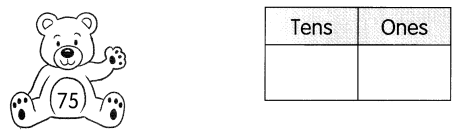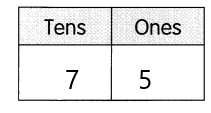# Math in Focus Grade 1 Chapter 16 Practice 2 Answer Key Place Value

Go through the Math in Focus Grade 1 Workbook Answer Key Chapter 16 Practice 2 Place Value to finish your assignments.

## Math in Focus Grade 1 Chapter 16 Practice 2 Answer Key Place Value

Look at the pictures. Then fill in the blanks.

Example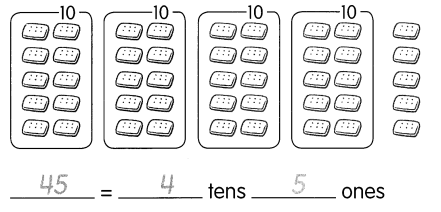Question 1.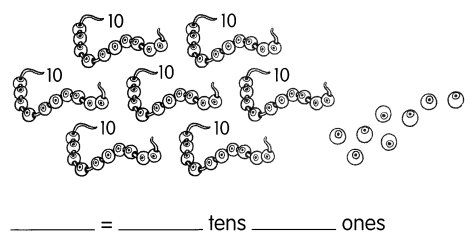78 = 7 tens 8 ones.

Explanation:
In the above image, we can see that there are 7 tens and 8 ones which are 78.

Question 2.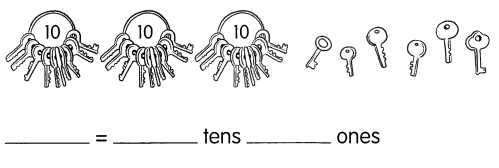36 = 3 tens 6 ones.

Explanation:
In the above image, we can see that there are 3 tens and 6 ones which are 36.

Question 3.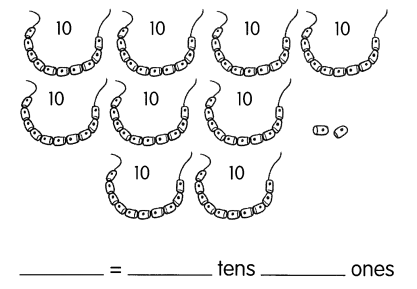92 = 9 tens 2 ones.

Explanation:
In the above image, we can see that there are 9 tens and 2 ones which are 92.

Question 4.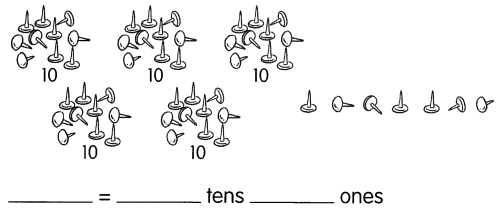57 = 5 tens 7 ones.

Explanation:
In the above image, we can see that there are 5 tens and 7 ones which are 57.

Question 5.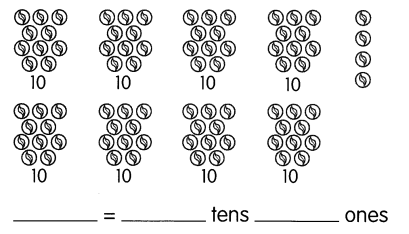84 = 8 tens 4 ones.

Explanation:
In the above image, we can see that there are 8 tens and 4 ones which are 84.

Count the base-ten blocks. Then fill in the blanks.

Example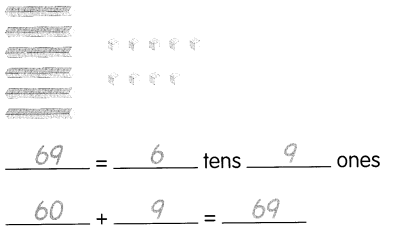Question 6.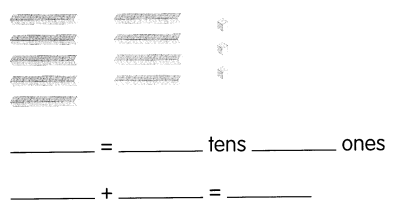93 = 9 tens 3 ones.
90 + 3 = 93.

Explanation:
In the above image, we can see that there are 9 tens and 3 ones which are 93.
90+3 = 93.

Question 7.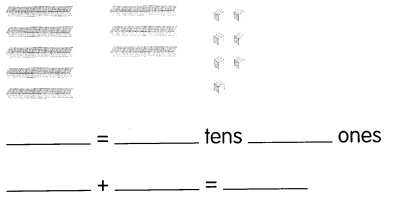87 = 8 tens 7 ones.
80+7 = 87.

Explanation:
In the above image, we can see that there are 8 tens and 7 ones which are 87.
80+7= 87.

Fill in the place-value charts.

Question 8.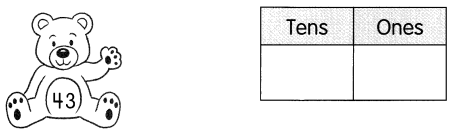43 = 4 tens 3 ones.

Explanation:
Here, place value is a value represented by a digit in a number on the basis of its position in the number. So the place value of 43 is 4 in the tens place and 3 in ones place.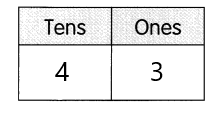Question 9.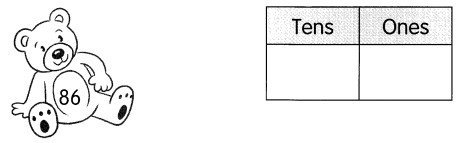86 = 8 tens and 6 ones.

Explanation:
Here, place value is a value represented by a digit in a number on the basis of its position in the number. So the place value of 86 is 8 in the tens place and 6 in ones place.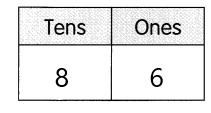Question 10.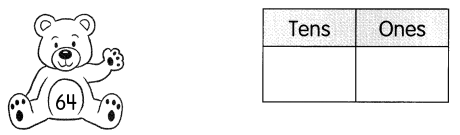64 = 6 tens 4 ones.

Explanation:
Here, place value is a value represented by a digit in a number on the basis of its position in the number. So the place value of 64 is 6 in the tens place and 4 in ones place.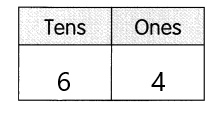Question 11.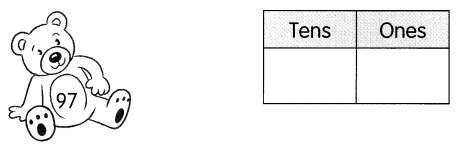97 = 9 tens 7 ones.

Explanation:
Here, place value is a value represented by a digit in a number on the basis of its position in the number. So the place value of 97 is 9 in the tens place and 7 in ones place.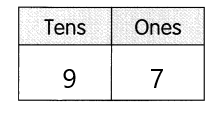Question 12.